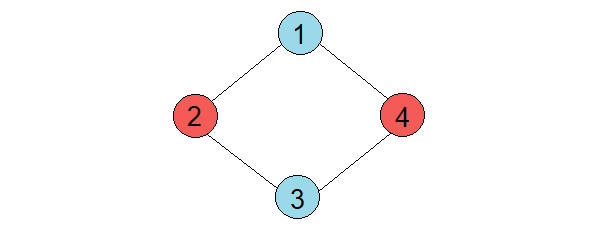Problem title
Difficulty
Avg time to solve

Detect Cycle In A Directed Graph
Moderate
30 mins
Smallest Range From K Sorted List
Moderate
15 mins
Longest Common Prefix After Rotation
Moderate
30 mins
Find Peak Element
Easy
15 mins
Square Submatrix with sum less than or equal to K
Moderate
30 mins
Weighted Job Scheduling
Moderate
15 mins
BST Iterator
Moderate
20 mins
Maximum Sum Increasing Subsequence
Moderate
10 mins
Find A Specific Pair In Matrix
Moderate
10 mins
Single Number
Easy
10 mins14

# Colour The Graph

Difficulty: MEDIUM
Avg. time to solve
20 min
Success Rate
80%

Problem Statement

#### You are given a graph with 'N' vertices numbered from '1' to 'N' and 'M' edges. You have to colour this graph in two different colours, say blue and red such that no two vertices connected by an edge are of the same colour.

##### Note :
``````The given graph may have connected components.
``````
##### Input Format :
``````The first line of input contains two integers 'V' and 'E', separated by a single space. They denote the total number of vertices and edges respectively.

From the second line onwards, the next 'E' lines represent an edge between the two vertices.

Every edge is represented by two vertices(u, v) that share an edge between them. The values of the vertices would again be separated by a single space.
``````
##### Output Format :
``````Print "YES" if it is possible else print "NO".
``````
##### Constraints :
``````1 <= E <= 10 ^ 5
1 <= V <= 10 ^ 6

Time Limit: 1 sec
``````
##### Sample Input 1 :
``````4 4
1 2
2 3
3 4
4 1
``````
##### Sample Output 1 :
``````YES
``````
##### Explanation for Sample 1 :
``````One possible coloring of the graph is:
``````##### Sample Input 2 :
``````3 3
1 2
2 3
3 1
``````
##### Sample Output 2 :
``````NO
``````Console